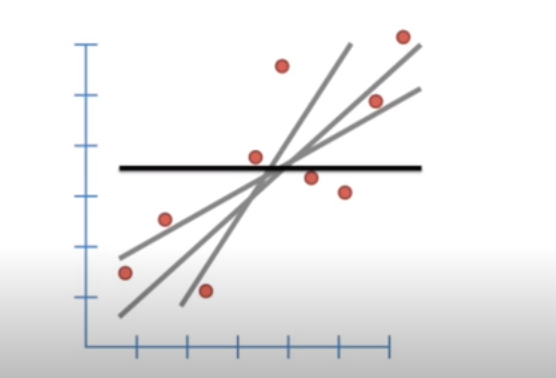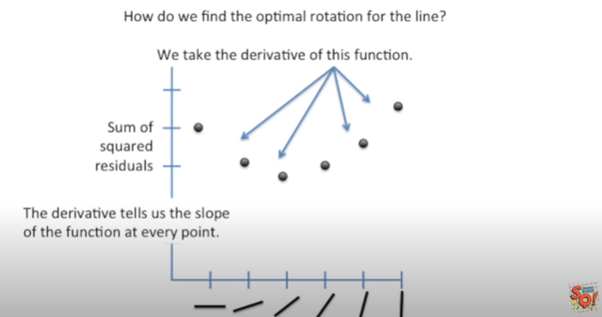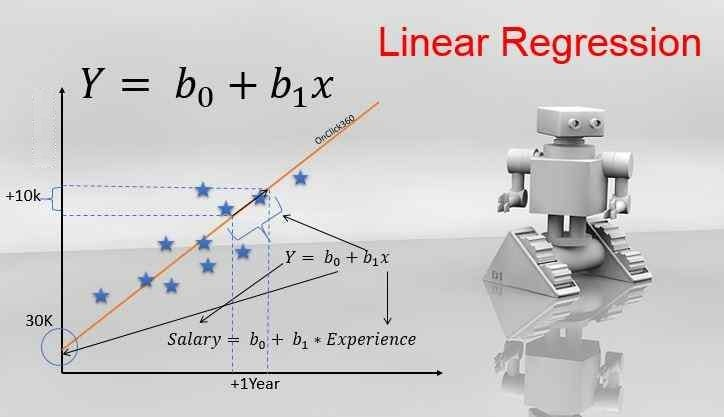1597760040

# Linear Regression and Fitting a Line to a data

Linear Regression is the Supervised Machine Learning Algorithm that predicts continuous value outputs. In Linear Regression we generally follow three steps to predict the output.

1. Use Least-Square to fit a line to data

2. Calculate R-Squared

3. Calculate p-value

## Fitting a line to a data

There can be many lines that can be fitted within the data, but we have to consider only that one which has very less error.Let say the bold line (‘b’), represents the average Y value and distance between b and all the datapoints known as residual.

(b-Y1) is the distance between b and the first datapoint. Similarly, (b-y2) and (b-Y3) is the distance between second and third datapoints and so on.

Note: Some of the datapoints are less than b and some are bigger so on adding they cancel out each other, therefore we take the squares of the sum of residuals.

SSR = (b-Y1)² + (b-Y2)² + (b-Y3)² + ………… + ……(b-Yn)² . where, n is the number of datapoints.

When for the line SSR is very less, the line is considered to be the best fit line. To find this best fit lines we need the help of equation of straight lines:

Y = mX+c

Where, m is the slope and c is the intercept through y_axis. Value of ‘m’ and ‘c’ should be optimal for SSR to be less.

SSR = ((mX1+c)-Y1)² + ((mX2+c)-Y2)² + ………. + …….

Where Y1, Y2, ……., Yn is the observed/actual value and,

(mX1+c), (mX2+c), ………. Are the value of line or the predicted value.

Since we want the line that will give the smallest SSR, this method of finding the optimal value of ‘m’ and ‘c’ is called Least-Square.Stat Quest

This is the plot of SSR versus Rotation of Lines. SSR goes down when we start rotating the line, and after a saturation point it starts increasing on further rotation. The line of rotation for which SSR is minimal is the best fitted line. We can use derivation for finding this line. On derivation of SSR, we get the slope of the function at every point, when at the point the slope is zero, the model select that line.

#hypothesis-testing #linear-regression #machine-learning #statistics #normal-distribution #testing